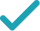Solve for aSteps Using the Quadratic Formula
Steps for Completing the Square
Solve for bSteps Using the Quadratic Formula
Steps for Completing the Square
Solve for RSolve for a (complex solution)Solve for b (complex solution)Still have questions?
a^{2}+3ba-b^{2}=R
All equations of the form ax^{2}+bx+c=0 can be solved using the quadratic formula: \frac{-b±\sqrt{b^{2}-4ac}}{2a}. The quadratic formula gives two solutions, one when ± is addition and one when it is subtraction.
a^{2}+3ba-b^{2}-R=R-R
Subtract R from both sides of the equation.
a^{2}+3ba-b^{2}-R=0
Subtracting R from itself leaves 0.
a=\frac{-3b±\sqrt{\left(3b\right)^{2}-4\left(-b^{2}-R\right)}}{2}
This equation is in standard form: ax^{2}+bx+c=0. Substitute 1 for a, 3b for b, and -b^{2}-R for c in the quadratic formula, \frac{-b±\sqrt{b^{2}-4ac}}{2a}.
a=\frac{-3b±\sqrt{9b^{2}-4\left(-b^{2}-R\right)}}{2}
Square 3b.
a=\frac{-3b±\sqrt{9b^{2}+4b^{2}+4R}}{2}
Multiply -4 times -b^{2}-R.
a=\frac{-3b±\sqrt{13b^{2}+4R}}{2}
a=\frac{\sqrt{13b^{2}+4R}-3b}{2}
Now solve the equation a=\frac{-3b±\sqrt{13b^{2}+4R}}{2} when ± is plus. Add -3b to \sqrt{4R+13b^{2}}.
a=\frac{-\sqrt{13b^{2}+4R}-3b}{2}
Now solve the equation a=\frac{-3b±\sqrt{13b^{2}+4R}}{2} when ± is minus. Subtract \sqrt{4R+13b^{2}} from -3b.
a=\frac{\sqrt{13b^{2}+4R}-3b}{2} a=\frac{-\sqrt{13b^{2}+4R}-3b}{2}
The equation is now solved.
a^{2}+3ba-b^{2}=R
Quadratic equations such as this one can be solved by completing the square. In order to complete the square, the equation must first be in the form x^{2}+bx=c.
a^{2}+3ba-b^{2}-\left(-b^{2}\right)=R-\left(-b^{2}\right)
Subtract -b^{2} from both sides of the equation.
a^{2}+3ba=R-\left(-b^{2}\right)
Subtracting -b^{2} from itself leaves 0.
a^{2}+3ba=b^{2}+R
Subtract -b^{2} from R.
a^{2}+3ba+\left(\frac{3b}{2}\right)^{2}=b^{2}+R+\left(\frac{3b}{2}\right)^{2}
Divide 3b, the coefficient of the x term, by 2 to get \frac{3b}{2}. Then add the square of \frac{3b}{2} to both sides of the equation. This step makes the left hand side of the equation a perfect square.
a^{2}+3ba+\frac{9b^{2}}{4}=b^{2}+R+\frac{9b^{2}}{4}
Square \frac{3b}{2}.
a^{2}+3ba+\frac{9b^{2}}{4}=\frac{13b^{2}}{4}+R
\left(a+\frac{3b}{2}\right)^{2}=\frac{13b^{2}}{4}+R
Factor a^{2}+3ba+\frac{9b^{2}}{4}. In general, when x^{2}+bx+c is a perfect square, it can always be factored as \left(x+\frac{b}{2}\right)^{2}.
\sqrt{\left(a+\frac{3b}{2}\right)^{2}}=\sqrt{\frac{13b^{2}}{4}+R}
Take the square root of both sides of the equation.
a+\frac{3b}{2}=\frac{\sqrt{13b^{2}+4R}}{2} a+\frac{3b}{2}=-\frac{\sqrt{13b^{2}+4R}}{2}
Simplify.
a=\frac{\sqrt{13b^{2}+4R}-3b}{2} a=\frac{-\sqrt{13b^{2}+4R}-3b}{2}
Subtract \frac{3b}{2} from both sides of the equation.
-b^{2}+3ab+a^{2}=R
All equations of the form ax^{2}+bx+c=0 can be solved using the quadratic formula: \frac{-b±\sqrt{b^{2}-4ac}}{2a}. The quadratic formula gives two solutions, one when ± is addition and one when it is subtraction.
-b^{2}+3ab+a^{2}-R=R-R
Subtract R from both sides of the equation.
-b^{2}+3ab+a^{2}-R=0
Subtracting R from itself leaves 0.
b=\frac{-3a±\sqrt{\left(3a\right)^{2}-4\left(-1\right)\left(a^{2}-R\right)}}{2\left(-1\right)}
This equation is in standard form: ax^{2}+bx+c=0. Substitute -1 for a, 3a for b, and a^{2}-R for c in the quadratic formula, \frac{-b±\sqrt{b^{2}-4ac}}{2a}.
b=\frac{-3a±\sqrt{9a^{2}-4\left(-1\right)\left(a^{2}-R\right)}}{2\left(-1\right)}
Square 3a.
b=\frac{-3a±\sqrt{9a^{2}+4\left(a^{2}-R\right)}}{2\left(-1\right)}
Multiply -4 times -1.
b=\frac{-3a±\sqrt{9a^{2}+4a^{2}-4R}}{2\left(-1\right)}
Multiply 4 times a^{2}-R.
b=\frac{-3a±\sqrt{13a^{2}-4R}}{2\left(-1\right)}
b=\frac{-3a±\sqrt{13a^{2}-4R}}{-2}
Multiply 2 times -1.
b=\frac{\sqrt{13a^{2}-4R}-3a}{-2}
Now solve the equation b=\frac{-3a±\sqrt{13a^{2}-4R}}{-2} when ± is plus. Add -3a to \sqrt{-4R+13a^{2}}.
b=\frac{-\sqrt{13a^{2}-4R}+3a}{2}
Divide -3a+\sqrt{-4R+13a^{2}} by -2.
b=\frac{-\sqrt{13a^{2}-4R}-3a}{-2}
Now solve the equation b=\frac{-3a±\sqrt{13a^{2}-4R}}{-2} when ± is minus. Subtract \sqrt{-4R+13a^{2}} from -3a.
b=\frac{\sqrt{13a^{2}-4R}+3a}{2}
Divide -3a-\sqrt{-4R+13a^{2}} by -2.
b=\frac{-\sqrt{13a^{2}-4R}+3a}{2} b=\frac{\sqrt{13a^{2}-4R}+3a}{2}
The equation is now solved.
-b^{2}+3ab+a^{2}=R
Quadratic equations such as this one can be solved by completing the square. In order to complete the square, the equation must first be in the form x^{2}+bx=c.
-b^{2}+3ab+a^{2}-a^{2}=R-a^{2}
Subtract a^{2} from both sides of the equation.
-b^{2}+3ab=R-a^{2}
Subtracting a^{2} from itself leaves 0.
\frac{-b^{2}+3ab}{-1}=\frac{R-a^{2}}{-1}
Divide both sides by -1.
b^{2}+\frac{3a}{-1}b=\frac{R-a^{2}}{-1}
Dividing by -1 undoes the multiplication by -1.
b^{2}+\left(-3a\right)b=\frac{R-a^{2}}{-1}
Divide 3a by -1.
b^{2}+\left(-3a\right)b=a^{2}-R
Divide R-a^{2} by -1.
b^{2}+\left(-3a\right)b+\left(-\frac{3a}{2}\right)^{2}=a^{2}-R+\left(-\frac{3a}{2}\right)^{2}
Divide -3a, the coefficient of the x term, by 2 to get -\frac{3a}{2}. Then add the square of -\frac{3a}{2} to both sides of the equation. This step makes the left hand side of the equation a perfect square.
b^{2}+\left(-3a\right)b+\frac{9a^{2}}{4}=a^{2}-R+\frac{9a^{2}}{4}
Square -\frac{3a}{2}.
b^{2}+\left(-3a\right)b+\frac{9a^{2}}{4}=\frac{13a^{2}}{4}-R